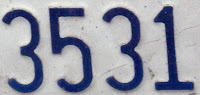## Friday, March 26, 2010

### 3531

3531 = 3 x 11 x 107.

3531 is a composite number whose three prime factors are distinct in length.

3531 is 24203 in base 6 and 13203 in base 7.

3531 is a multiple of 11 having only odd digits.

3531 is the number of sums payable using exactly 39 banknotes of denominations 1, 5, 10, 20, 50, and 100. For n = 1, there are precisely six payable sums.The Lego 3531 set was a kit for building the Znap Tri-bike.